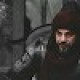Home » Forces » Non Verbal Test » Non Verbal Test Online Aptitude Classification Quiz 1

# Non Verbal Test Online Aptitude Classification Quiz 1

Given on this page is a free online Non Verbal Aptitude Classification test through which all the individuals who are preparing for any kind of exam such as aptitude or armed forces like Army, Navy and Air Force, can attempt this quiz in order to prepare for it with ease. Our quizzes will help you in not only evaluating your current level of preparation but also improving it.

## Non Verbal

1. Out of the given figure, four are similar in a certain way. One figure is not like the other four. That means four figure from a group. The question in which one of the figures does not belong to this group?Question 1 of 7

2. Out of the given figure, four are similar in a certain way. One figure is not like the other four. That means four figure from a group. The question in which one of the figures does not belong to this group?Question 2 of 7

3. Out of the given figure, four are similar in a certain way. One figure is not like the other four. That means four figure from a group. The question in which one of the figures does not belong to this group?Question 3 of 7

4. Out of the given figure, four are similar in a certain way. One figure is not like the other four. That means four figure from a group. The question in which one of the figures does not belong to this group?Question 4 of 7

5. Out of the given figure, four are similar in a certain way. One figure is not like the other four. That means four figure from a group. The question in which one of the figures does not belong to this group?Question 5 of 7

6. Out of the given figure, four are similar in a certain way. One figure is not like the other four. That means four figure from a group. The question in which one of the figures does not belong to this group?Question 6 of 7

7. Out of the given figure, four are similar in a certain way. One figure is not like the other four. That means four figure from a group. The question in which one of the figures does not belong to this group?Question 7 of 7

You Can Learn and Gain more Knowledge through our Online Quiz and Testing system Just Search your desired Preparation subject at Gotest.

1.In question no 6(b) option is correct because right wing is shaded

•yes i also mark b

2.71%

3.4.5.Awais UR Rehman Hiraj

b is correct

6.I am also thinking that (B) would be correct

•its d because a and e are same b and c are same different is d

•amazing observation

error: